Courses

# Ex 3.2 NCERT Solutions- Pair of Linear Equations in Two Variables Class 10 Notes | EduRev

## Class 10 : Ex 3.2 NCERT Solutions- Pair of Linear Equations in Two Variables Class 10 Notes | EduRev

The document Ex 3.2 NCERT Solutions- Pair of Linear Equations in Two Variables Class 10 Notes | EduRev is a part of the Class 10 Course Class 10 Mathematics by VP Classes.
All you need of Class 10 at this link: Class 10

Ques 1: Form the pair of linear equations in the following problems, and find their solutions graphically.
(i) 10 students of Class X took part in a Mathematics quiz. If the number of girls is 4 more than the number of boys, find the number of boys and girls who took part in the quiz.
(ii) 5 pencils and 7 pens together cost ₹ 50, whereas 7 pencils and 5 pens together cost ₹ 46. Find the cost of one pencil and that of one pen.
Sol: (i) Let The number of boys = x
∴ Number of girls = y
Number of students = 10
∴ x + y = 10 ...(1)
∴ Number girls = [Number of boys] + 4
∴ y = x + 4
⇒ x - y = - 4 ...(2)
∴ Required pair of equations is x + y = 10; x - y = - 4.
Now, from the equation (1), we have
y = 10 - x
∴ Table of solution for equation (1),

 x 6 4 5 y 4 6 5 (x, y) (6, 4) (4, 6) (5, 5)

And from the equation (2), we have
y = x + 4
∴ Table of solution for equation (2),

 x 0 1 2 y 4 5 6 (x, y) (0, 4) (1, 5) (2, 6)

Now plotting the points and drawing the lines.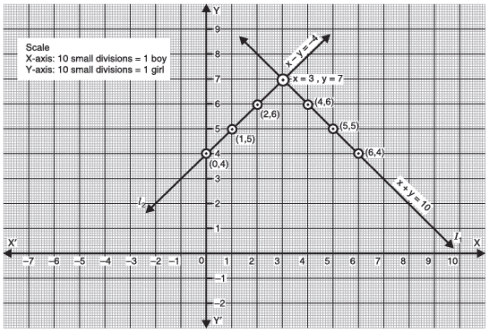As l1 and l2 intersect at the point (3, 7),
∴ The solution of the pair of linear equations is: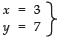∴ Required number of boys = 3
Required number of girls = 7
(ii) Let the cost of a pencil = ₹ x
And cost of a pen = ₹ y
Since cost of 5 pencils + Cost of 7 pens = ₹ 50
⇒ 5x + 7y = 50 ...(1)
Also cost of 7 pencils + cost of 5 pens = ₹ 46
⇒ 7x + 5y = 46 ...(2)
Thus, the required pair of equations is: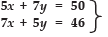Now, from 5x + 7y = 50, we have:

 x 10 3 -4 y 0 5 10 (x, y) (10, 0) (3, 5) (-4, 10)

And from 7x + 5y = 46, we have:

 x 8 3 0 y -2 5 9.2 (x, y) (8, -2) (3, 5) (0, 9.2)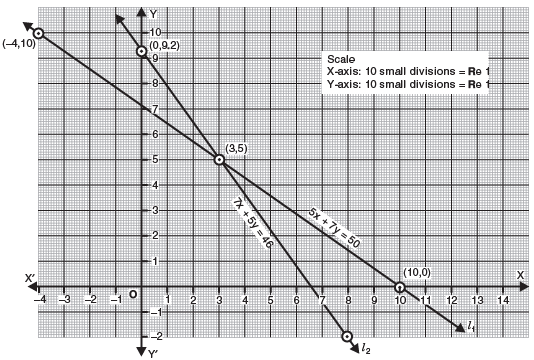Plotting the points (10, 0) (3, 5) and (- 4, 10) we get a straight line l1 as the graph of 5x + 7y = 50, and plotting the points (8, - 2), (3, 5) and (10, 0), we get a straight line l2 as the graph 7x + 5y = 46.
The two straight lines intersect at (3, 5)
∴ Cost of a pencil = ₹ 3
and Cost of a pen = ₹ 5

Ques 2: On comparing the ratios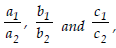, find out whether the lines representing the following pairs of linear equations intersect at a point, are parallel or coincident:
(i) 5x - 4y + 8 = 0, 7x + 6y - 9 = 0
(ii) 9x + 3y + 12 = 0, 18x + 6y + 24 = 0
(iii) 6x - 3y + 10 = 0, 2x - y + 9 = 0
Sol: Comparing the given equations with
a1 x + b1 y + c1 = 0
a2 x + b2 y + c2 = 0
We have,
(i) For, 5x - 4y + 8 = 0
7x + 6y - 9 = 0
a1 = 5, b1 = - 4, c1 = 8
a2 = 7, b2 = 6, c2 = - 9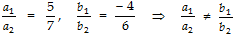∴ Their graph lines are intersecting, i.e. the intersect at a point.
(ii) For, 9x + 3y + 12 = 0
18x + 6y + 24 = 0
a1 = 9  b1 = 3  c1 = 12
a2 = 18  b2 = 6  c2 = 24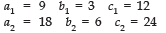and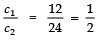i.e.,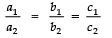Their graph lines are coincident.

(iii) For, 6x - 3y + 10 = 0
2x - y + 9 = 0
a= 6,  b1 = - 3,  c1 = 10
a2 = 2,  b2 = - 1,  c2 = 9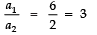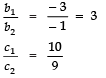and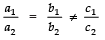⇒ Their graph lines are parallel.

Ques 3: On comparing the ratios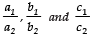find out whether the following pairs of linear equations are consistent, or inconsistent.
(i) 3x + 2y = 5;  2x - 3y = 7

(ii) 2x - 3y = 8;  4x - 6y = 9
(iii)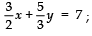9x - 10y = 14

(iv) 5x - 3y = 11;  - 10x + 6y = - 22
(v)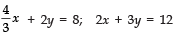Sol: Comparing the given pairs of linear equations with the standard pair of linear equations,
a1x + b1y + c1 = 0
a2x + b2y + c2 = 0
We have:
(i) For 3x + 2y = 5
2x - 3y = 7
a1 = 3,   b1 = 2,   c1 = 5
a= 2,   b2 = - 3,  c2 = 7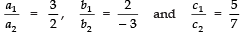Since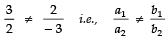∴ Their graph lines are intersecting at a point.
⇒ The given pair is consistent.
(ii)  For  2x - 3y  =  8
4x - 6y  =  9
a1 = 2,  b1 = - 3,  c1 = 8
a2 = 4,   b2 = - 6,  c2 = 9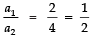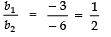and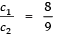Here,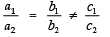⇒ Their graph lines are parallel i.e., the given pair of linear equations has no solution.
∴ It is a pair of inconsistent equations.
(iii) For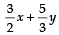=  7
9x - 10y = 14
a1 = 3/2,  b1 = 5/3,  c1 = 7
a2 = 9, b2 = - 10,  c2 = 14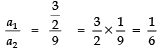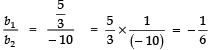⇒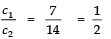Here,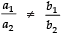⇒ Their graphs are intersecting.
∴ The given pair of linear equations has one solution.
⇒ It is a pair of consistent equations.
(iv) For  5x - 3y  =  11
- 10x + 6y  =  - 22
a1 = 5,  b1 = - 3,  c1 = 11
a2 = - 10, b2 = 6,  c= - 22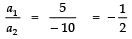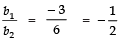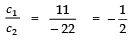Here,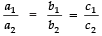∴ Their graph lines are coincident.
⇒ The given pair of linear equations has infinitely many solutions.
Thus, they are consistent.

(v) For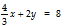= 8
2x + 3y = 12
a1 = 4/3,  b1 = 2,  c1 = 8
a2 = 2,  b2 = 3,  c2 = 12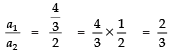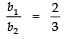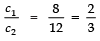Since =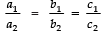∴ Their graphs are consistent i.e., they have infinitely many solutions.
⇒ The given pair of linear equations is consistent.

Ques 4: Which of the following pairs of linear equations are consistent/inconsistent? If consistent, obtain the solution graphically:
(i) x + y = 5,  2x + 2y = 10
(ii) x - y = 8,  3x - 3y = 16
(iii) 2x + y - 6 = 0,  4x - 2y - 4 = 0
(iv) 2x - 2y - 2 = 0,  4x - 4y - 5 = 0
Sol: (i) For x + y = 5
2x + 2y = 10
We have a1 = 1,  b1 = 1,  c1 = 5
a2 = 2,  b2 = 2,  c2 = 10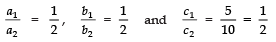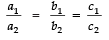∴ Their graph lines are coincident, as shown
x + y  =  5

 x 0 5 1 y 5 0 4 (x, y) (0, 5) (5, 0) (1, 4)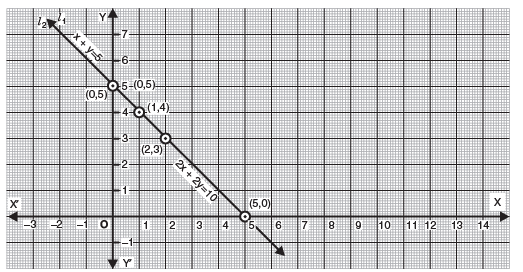2x + 2y = 10

 x 2 0 5 y 3 5 0 (x, y) (2, 3) (0, 5) (5, 0)

The graphs i.e., l1 and l2 are coinciding.
i.e. They have infinitely many solutions.
(ii)  For  x - y  =  8
3x - 3y  =  16
∵  a =  1,  b1 = - 1  and  c1 = 8
a=   3,  b2 = - 3  and  c2 = 16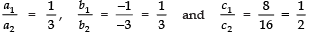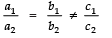∴ The pair of linear equations is inconsistent.
(iii)  For  2x + y - 6  =  0
4x - 2y - 4  =  0
⇒ a1  =  2,  b1 = 1  and  c1 = - 6
a2  =  4,  b2 = - 2,  and  c2 = - 4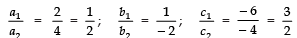Here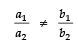⇒ Constistent pair of linear equations.
Graphical Solution
2x + y − 6 = 0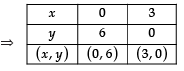and 4x − 2y − 4 = 0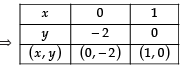Plotting the points (0, 6), (3, 0), we get a straight line l1
Plotting the points (0, − 2), (1, 0), we get a straight line l2
∵ l1 and l2 intersect at (2, 2)
∴ x = 2 and y = 2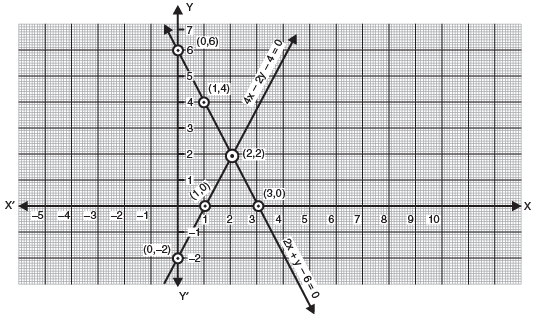(iv) For 2x - 2y - 2 = 0
4x - 4y - 5 = 0
a1 = 2,  b1 = - 2,  c1 = - 2
a2 = 4,  b2 = - 4,  c2 = - 5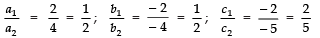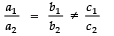i.e., the given pair of linear equations is inconsistent.

Ques 5: Half the perimeter of a rectangular garden, whose length is 4 m more than its width, is 36 m. Find the dimensions of the garden.
Sol: Let the width of the garden = x m
And the length of the garden = y m
∴ Condition I, gives
4 + width = length
⇒ 4 + x = y
⇒ y - x = 4   ...(1)
Also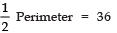y + x =  36  ...(2)
Graphical Solution:
y - x = 4

 x 0 -4 1 y 4 0 5 (x, y) (0, 4) (-4, 0) (1, 5)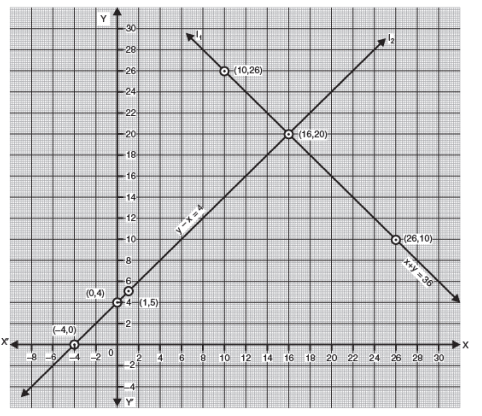and  x + y = 36

 x 10 26 16 y 26 10 20 (x, y) (10, 26) (10, 26) (16, 20)

The lines l1 and l2 intersect at (16, 20).
∴  x = 16  and  y = 20
i.e., Length = 20 m

Ques 6: Given the linear equation 2x + 3y - 8 = 0, write another linear equation in two variables such that the geometrical representation of the pair so formed is:
(i) intersecting lines
(ii) parallel lines
(iii) coincident lines
Sol: (i) Let the pair of linear equations be
2x + 3y - 8 = 0 ⇒ a1 = 2,  b1 = 3  and  c1 = - 8
a2x + b2y + c2 = 0
For intersecting lines, we have: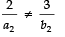∴ We can have
a2 = 3,  b = 2  and  c2 = - 7
∴ The required equation can be
3x + 2y - 7 = 0

Note:
There can be many such equations.

(ii) For parallel lines,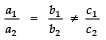∴ Any line parallel to 2x + 3y - 8 = 0, can be taken as
2x + 3y - 12 = 0
(iii) For coincident lines,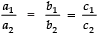∴ Any line parallel to 2x + 3y - 8 = 0 can be 2 (2x + 3y - 8 = 0) i.e.
4x + 6y - 16 = 0

Ques 7: Draw the graphs of the equations x - y + 1 = 0 and 3x + 2y - 12 = 0. Determine the coordinates of the vertices of the triangle formed by these lines and the x-axis, and shade the triangular region.
Sol: x - y + 1 = 0
3x + 12y - 12 = 0
To draw the graphs of the above given pair of linear equations,
x - y + 1 = 0

 x 2 -1 3 y 3 0 4 (x, y) (2, 3) (-1, 0) (3, 4)

Plotting, the points (2, 3), (- 1, 0) and (3, 4) we get a straight line l1.
Also
3x + 2y - 12 = 0

 x 2 4 0 y 3 0 6 (x, y) (2, 3) (4, 0) (0, 6)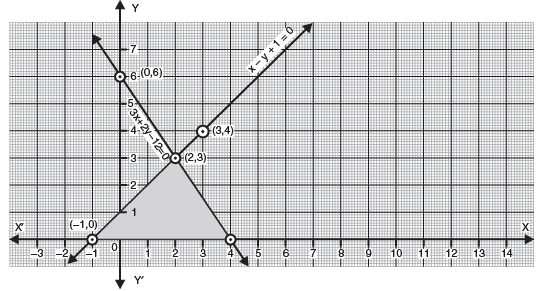Plotting the points (2, 3), (4, 0) and (0, 6), we get a straight line l2.
The lines l1 and l2 intersect at (2, 3). The line l1 intersect the x-axis at (4, 0) and the line l2 intersect the x-axis at (- 1, 0).
Thus, coordinates of the vertices of the shaded triangular region are (4, 0), (- 1, 0) and
(2, 3).
Algebraic Method of Solving a Pair of Linear Equations
1. Substitution Method
For solving two equations in x and y simultaneously, we proceed according to the following steps:
I. Express one of the variables in terms of the other.
II. Substitute this value in the other equation and get a linear equation in the other variable.
III. Solve this equation and find the value of the other variable.
IV. Substitute this value in any one of the given equations and find the value of the first variable.

Offer running on EduRev: Apply code STAYHOME200 to get INR 200 off on our premium plan EduRev Infinity!

132 docs

,

,

,

,

,

,

,

,

,

,

,

,

,

,

,

,

,

,

,

,

,

;